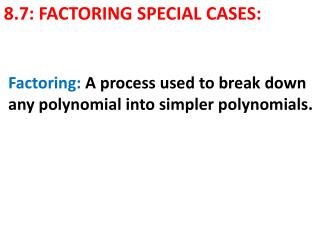# 8.7: FACTORING SPECIAL CASES: - PowerPoint PPT PresentationDownload Presentation8.7: FACTORING SPECIAL CASES:

8.7: FACTORING SPECIAL CASES:Download Presentation## 8.7: FACTORING SPECIAL CASES:

- - - - - - - - - - - - - - - - - - - - - - - - - - - E N D - - - - - - - - - - - - - - - - - - - - - - - - - - -
##### Presentation Transcript

1. Factoring: A process used to break down any polynomial into simpler polynomials. 8.7: FACTORING SPECIAL CASES:

2. Procedure: 1) Always look for the GCF of all the terms FACTORING ax2 + bx + c 2) Factor the remaining terms – pay close attention to the value of coefficient a and follow the proper steps. 3) Re-write the original polynomial as a product of the polynomials that cannot be factored any further.

3. Case 1: (a+b)2 ↔ (a+b)(a+b) ↔ a2 +ab+ab+b2 ↔ a2+2ab+b2 FACTORING : Case 2: (a-b)2 ↔ (a-b)(a-b) ↔ a2 –ab-ab+b2 ↔ a2-2ab+b2 Case 3: (a+b)(a-b) ↔ a2 +ab-ab-b2 ↔ a2- b2

4. GOAL:

5. FACTORING: A perfect square trinomial Ex: What is the FACTORED form of: x2-18x+81?

6. SOLUTION: since the coefficient is 1, we follow the process same process: x2-18x+81 ax2+bx+c  b= -18  c = +81 Look at the factors of c:  c = +81 : (1)(81), (-1)(-81) (9)(9), (-9)(-9) Take the pair that equals to b when adding the two integers. We take (-9)(-9) since -9+ -9 = -18= b Factored form : (x-9)(x-9) = (x-9)2

7. YOU TRY IT: Ex: What is the FACTORED form of: x2+6x+9?

8. SOLUTION: since the coefficient is 1, we follow the process same process: X2+6x+9 ax2+bx+c  b= +6  c = +9 Look at the factors of c:  c = +9 : (1)(9), (-1)(-9) (3)(3), (-3)(-3) Take the pair that equals to b when adding the two integers. We take (3)(3) since 3+3 = +6 = b Factored form : (x+3)(x+3) = (x+3)2

9. FACTORING: A Difference of Two Squares Ex: What is the FACTORED form of: z2-16?

10. SOLUTION: since there is no b term, then b = 0 and we still look at c: z2-16 az2+bz+c  b= 0  c = -16 Look at the factors of c:  c = -16 : (-1)(16), (1)(-16) (-2)(8), (2)(-8), (-4)(4) Take the pair that equals to b when adding the two integers. We take (-4)(4) since 3-4 = 0 = b Thus Factored form is : (z-4)(z+4)

11. YOU TRY IT: Ex: What is the FACTORED form of: 16x2-81?

12. SOLUTION: To factor a difference of two squares with a coefficient ≠ 1 in the x2, we must look at the a and c coefficients: 16x2-81 ax2+c  a= +16  c =-81 Look at the factors of a and c: a : (4)(4) c: (-9)(9) We now see that the factored form is: (4x-9)(4x+9)

13. YOU TRY IT: Ex: What is the FACTORED form of: 24x2-6?

14. SOLUTION: To factor a difference of two squares with a coefficient ≠ 1 we still follow the factoring procedure: 24x2-6  6(4x2-1) ax2+c  a= +4  c =-1 Look at the factors of a and c: a : (2)(2) c: (-1)(1) We now see that the factored form is: 6(2x-1)(2x+1)

15. REAL-WORLD: The area of a square rug is given by 4x2-100.What are the possible dimensions of the rug?

16. SOLUTION: To factor a difference of two squares with a coefficient ≠ 1 we still follow the factoring procedure: 4x2-100  4(x2-25) ax2+c  a= +1  c =-25 Look at the factors of a and c: a : (1)(1) c: (-5)(5) We now see that the factored form is: 4(x-5)(x+5)

17. VIDEOS: FactoringQuadratics Factoring with GCF: http://www.khanacademy.org/math/algebra/quadratics/factoring_quadratics/v/factoring-trinomials-with-a-common-factor Factoring perfect squares: http://www.khanacademy.org/math/algebra/quadratics/factoring_quadratics/v/factoring-perfect-square-trinomials

18. VIDEOS: FactoringQuadratics Factoring with GCF: http://www.khanacademy.org/math/algebra/quadratics/factoring_quadratics/v/u09-l2-t1-we1-factoring-special-products-1

19. CLASSWORK:Page 514-516: Problems: 1, 2, 3, 9, 13, 16, 22, 27, 30, 32, 37, 45.